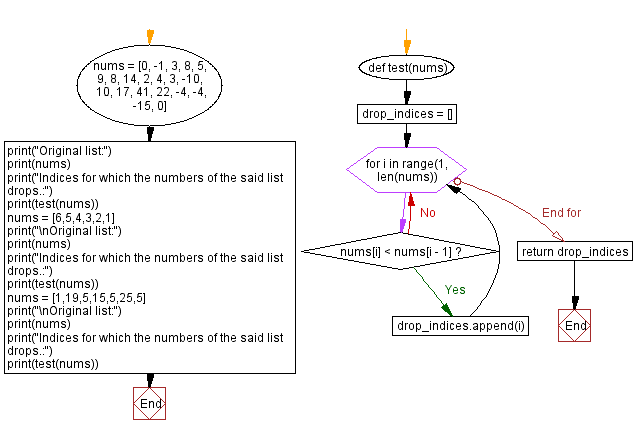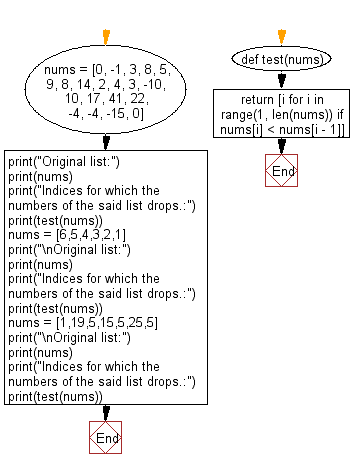﻿ Python: Find the indices for which the numbers in the list drops - w3resource# Python: Find the indices for which the numbers in the list drops

## Python Programming Puzzles: Exercise-23 with Solution

Write a Python program to find the indices for which the numbers in the list drops.

NOTE: You can detect multiple drops just by checking if nums[i] < nums[i-1].

```Input:
[0, -1, 3, 8, 5, 9, 8, 14, 2, 4, 3, -10, 10, 17, 41, 22, -4, -4, -15, 0]
Output:
[1, 4, 6, 8, 10, 11, 15, 16, 18]

Input:
[6, 5, 4, 3, 2, 1]
Output:
[1, 2, 3, 4, 5]

Input:
[1, 19, 5, 15, 5, 25, 5]
Output:
[2, 4, 6]
```

Sample Solution-1:

Python Code:

``````#License: https://bit.ly/3oLErEI

def test(nums):
drop_indices = []
for i in range(1, len(nums)):
if nums[i] < nums[i - 1]:
drop_indices.append(i)
return drop_indices
nums = [0, -1, 3, 8, 5, 9, 8, 14, 2, 4, 3, -10, 10, 17, 41, 22, -4, -4, -15, 0]
print("Original list:")
print(nums)
print("Indices for which the numbers of the said list drops.:")
print(test(nums))
nums = [6,5,4,3,2,1]
print("\nOriginal list:")
print(nums)
print("Indices for which the numbers of the said list drops.:")
print(test(nums))
nums = [1,19,5,15,5,25,5]
print("\nOriginal list:")
print(nums)
print("Indices for which the numbers of the said list drops.:")
print(test(nums))
``````

Sample Output:

```Original list:
[0, -1, 3, 8, 5, 9, 8, 14, 2, 4, 3, -10, 10, 17, 41, 22, -4, -4, -15, 0]
Indices for which the numbers of the said list drops.:
[1, 4, 6, 8, 10, 11, 15, 16, 18]

Original list:
[6, 5, 4, 3, 2, 1]
Indices for which the numbers of the said list drops.:
[1, 2, 3, 4, 5]

Original list:
[1, 19, 5, 15, 5, 25, 5]
Indices for which the numbers of the said list drops.:
[2, 4, 6]
```

Flowchart:## Visualize Python code execution:

The following tool visualize what the computer is doing step-by-step as it executes the said program:

Sample Solution-2:

Python Code:

``````#License: https://bit.ly/3oLErEI

def test(nums):
return [i for i in range(1, len(nums)) if nums[i] < nums[i - 1]]

nums = [0, -1, 3, 8, 5, 9, 8, 14, 2, 4, 3, -10, 10, 17, 41, 22, -4, -4, -15, 0]
print("Original list:")
print(nums)
print("Indices for which the numbers of the said list drops.:")
print(test(nums))
nums = [6,5,4,3,2,1]
print("\nOriginal list:")
print(nums)
print("Indices for which the numbers of the said list drops.:")
print(test(nums))
nums = [1,19,5,15,5,25,5]
print("\nOriginal list:")
print(nums)
print("Indices for which the numbers of the said list drops.:")
print(test(nums))
``````

Sample Output:

```Original list:
[0, -1, 3, 8, 5, 9, 8, 14, 2, 4, 3, -10, 10, 17, 41, 22, -4, -4, -15, 0]
Indices for which the numbers of the said list drops.:
[1, 4, 6, 8, 10, 11, 15, 16, 18]

Original list:
[6, 5, 4, 3, 2, 1]
Indices for which the numbers of the said list drops.:
[1, 2, 3, 4, 5]

Original list:
[1, 19, 5, 15, 5, 25, 5]
Indices for which the numbers of the said list drops.:
[2, 4, 6]
```

Flowchart:## Visualize Python code execution:

The following tool visualize what the computer is doing step-by-step as it executes the said program:

Python Code Editor :

Have another way to solve this solution? Contribute your code (and comments) through Disqus.

What is the difficulty level of this exercise?

Test your Programming skills with w3resource's quiz.

﻿

## Python: Tips of the Day

Clamps num within the inclusive range specified by the boundary values x and y:

Example:

```def tips_clamp_num(num,x,y):
return max(min(num, max(x, y)), min(x, y))
print(tips_clamp_num(2, 4, 6))
print(tips_clamp_num(1, -1, -6))
```

Output:

```4
-1
```